# Reverse Number Signs

This post will guide you how to reverse number signs in Excel. How do I reverse the sign of a range of cells from positive to negative or negative to positive in Excel. How to reverse sign of number with a formula in Excel.

Assuming that you have a list of data in range B1:B4 that contain some numbers, and you want to reverse the sign of those numbers. You can use the below two methods to achieve it.

## Method1: Reverse Number Signs with a Formula

You can multiply all numbers in a range of cells by a negative number 1, so that you can get the expected value. So just type the following formula in a blank cell C1.

`=B1*-1`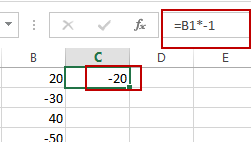Then you need to drag the AutoFill Handle over other cells to apply this formula.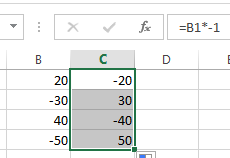So the positive number will be converted to negative numbers and the negative number will be converted to positive numbers. Then you still need to copy and paste the last result to the original range of cells.

## Method2: Reverse Number Signs with Paste Special Feature

You can also use the Paste Special Feature to reverse number signs in Excel. Just do the following steps:

#1 type negative number -1 in a blank cell and press Ctrl+C to copy it.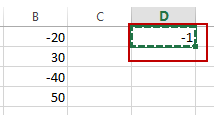#2 select the range of cells that contain numbers you want to reverse the signs. And right click on it and select Paste Special from the popup menu list. And the Paste Special dialog will open.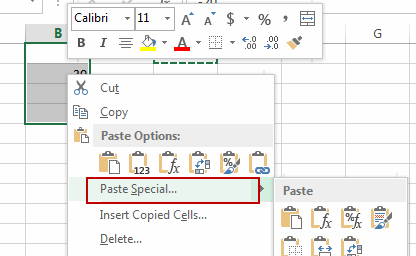#3 select Multiply radio button in the Operation section. And then click OK button.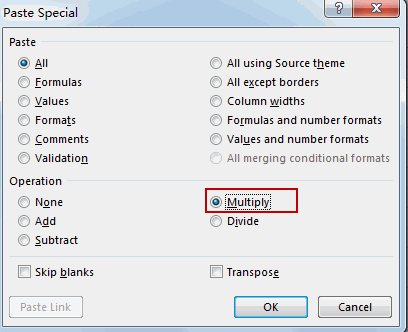#4 you will see that all of the selected cells will be multiplied by negative -1. And all number signs will be reversed.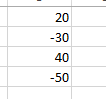Related Posts
If Cell is This Value or That Value

IF function is frequently used in Excel worksheet to return you expect “true value” or “false value” based on the result of logical test. If you want to see if a cell is A or B, and if one of ...

If Value is Greater Than A Certain Value

IF function is frequently used in Excel worksheet to return you expect “true value” or “false value” based on the logical test result. If you want to see if a value in one cell is greater than a specific value, ...

If Cell is Not Blank

IF function is frequently used in Excel worksheet to return you expect “true value” or “false value” based on the result of created logical test. If you want to see if a cell is blank or not, and leave some ...

VBA Macro For VLOOKUP From Another Sheet

In the previous post, you should know that how to fix or remove the #N/A error when using VLOOKUP formula to lookup value from another sheet. And this post will show you how to use VBA code to vlookup data ...

If Cell is Blank

IF function is frequently used in Excel worksheet to return you expect “true value” or “false value” based on the result of created logical test. If you want to see if a cell is blank or not, and leave some ...

If Cell Equals Certain Text String

IF function is frequently used in Excel worksheet to return you expect “true value” or “false value” based on the result of created logical test. If you want to see if cell equals a certain text string like “Win”, you ...

If Cell Contains Either Text1 or Text2

IF function is frequently used in Excel worksheet to return “true value” or “false value” based on the logical test result. If you want to see if cell contains certain substring1 like “abc” or substring2 like “def”, and returns true ...

If Cell Contains Certain Text OR Equals Certain Text

IF cell equals certain text IF function is frequently used in Excel worksheet to return “true value” or “false value” based on the logical test result. If you want to test values to see if they equal certain text like ...

VLOOKUP From Another Sheet Not Working

In the previous post, you should know that how to fix or remove the #N/A error when using VLOOKUP formula to lookup value from another sheet. And this post will show you reasons why your VLOOKUP formula is not working ...

If Cell Begins with One of Three Supplied Characters

If you want to test values to see if they begin with some given specific characters like “x”, ”y”, or “z”, you can create a formula with COUNTIF and SUM functions to return results. EXAMPLE You can see “TRUE” or ...

Sidebar